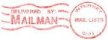McXtrace: Source_div

# The Source_div Component

X-ray source with Gaussian or uniform divergence

## Identification

• Site:
• Author: Erik Knudsen
• Origin: Risoe
• Date: November 11, 2009

## Description

A flat rectangular surface source with uniform or Gaussian divergence profile and focussing.
If the parametere gauss is not set (the default) the divergence profile is flat
in the range [-focus_ax,focus_ay]. If gauss is set, the focux_ax,focus_ay is considered
the standard deviation of the gaussian profile.
Currently focussing is only active for flat profile. The "focus window" is defined by focus_xw,focus_yh and dist.
The spectral intensity profile is uniformly distributed in the energy interval defined by e0+-dE/2 or
by wavelength lambda0+-dlambda/2

Example: Source_div(xwidth=0.1, yheight=0.1, focus_aw=2, focus_ah=2, E0=14, dE=2, gauss=0)

%VALIDATION

%BUGS


## Input parameters

Parameters in boldface are required; the others are optional.
 Name Unit Description Default spectrum_file File from which to read the spectral intensity profile NULL xwidth m Width of source. 0 yheight m Height of source. 0 focus_aw rd Standard deviation (Gaussian) or maximal (uniform) horz. width divergence. 0 focus_ah rd Standard deviation (Gaussian) or maximal (uniform) vert. height divergence. 0 focus_ar 0 radius 0 E0 keV Mean energy of X-rays. 0 dE keV Energy half spread of X-rays. If gauss==0 dE is the half-spread, i.e. E\in[E0-dE,E0+dE], if gauss!=0 it's interpreted as the standard dev. 0 lambda0 AA Mean wavelength of X-rays (only relevant for E0=0). 0 dlambda AA Wavelength half spread of X-rays. 0 flux 1/(s * mm**2 *mrad**2 * energy unit ) flux per energy unit, Angs or keV. 0 gauss 1 Criterion: 0: uniform, 1: Gaussian distribution of energy/wavelength. 0 gauss_a 1 Criterion: 0: uniform, 1: Gaussian divergence distribution. 0 randomphase 1 phase 0

## Output parameters

Parameters in boldface are required; the others are optional.
 Name Unit Description Default prms p_init dist